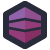Gary inherited some C code. This C code is correct, and does what it is supposed to, which is itself a pretty straightforward problem: create an array of values from 0-N, arranged in a random order.

Now, there are a lot of possible ways to do this, most of which involve swapping cells in the array around. It's quick, efficient, and easy to understand. Which means Gary's co-worker needed to find a better solution.

``````typedef struct {
int value;
double weight;
} valweight_t;

int f_cmp_valweight(const void * pv1, const void *pv2)
{
const valweight_t *p1 = (const valweight_t*) pv1;
const valweight_t *p2 = (const valweight_t*) pv2;

if (p1->weight > p2->weight) {
return 1;
} else if (p1->weight < p2->weight) {
return -1;
} else {
return 0;
}
}

void randomperm(int *a, int nb)
{
int i;
valweight_t *aValWeight = (valweight_t*) malloc(nb*sizeof(valweight_t));

for(i=0; i<nb; i++) {
aValWeight[i].value = i;
int n = rand() % nb;
double weight = ((double) n) / ((double) nb);
aValWeight[i].weight = weight;
}

qsort(aValWeight, nb, sizeof(valweight_t), f_cmp_valweight);

for(i=0; i<nb; i++) {
a[i] = aValWeight[i].value;
}

free(aValWeight);
}
``````

Let's trace through this. First, we create a new typ4e, `valweight_t`. It's a pair of an integer and a double.

Then we have `f_cmp_valweight`. This is a pretty straightforward comparison function, that converts some `void` pointers into `valweight_t`s and compares their weights.

The meat of this algorithm is `randomperm`, which is supposed to select a random permutation of a sequence of integers. `randomperm` takes a pointer to a bunch of `int`s, and a number of `int`s it should generate.

We start by creating an equally sized array of `valweight_t` structs. Then we populate that array- the `value` of each item is just the loop counter, and the weight is essentially a random number in the range 0-1.

We then quicksort that array, using that `f_cmp_valweight` function. Finally, we copy the values over into the input array parameter.

Now, standing alone, this set of functions is just… hideous. It's a massively overcomplicated solution for solving what should be an incredibly simple problem. Doing this with a Fisher-Yates shuffle would avoid extra memory allocations, avoid the need for an extra type, and just be the standard way of doing this. Plus, we wouldn't need to quicksort, which I assume this isn't generating incredibly large lists, but still- avoiding an unnecessary sort to just generate a random sequence seems better.

But Gary adds some extra context to this code, which reveals some true horrors lurking below:

The very same algorithm-savvy persons also wrote the compiler and a whole lot of tree-exploring garbage I have the misfortune of maintaining.

The people who wrote this code also wrote a custom compiler? Oh no. Oh no.[Advertisement] Continuously monitor your servers for configuration changes, and report when there's configuration drift. Get started with Otter today!